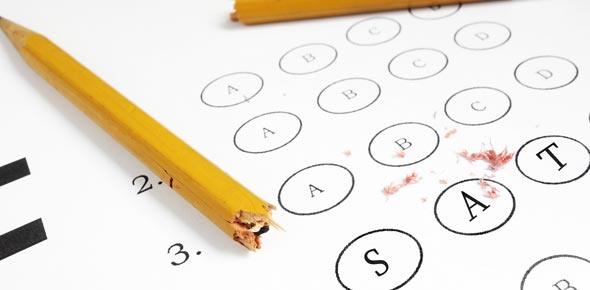# Can You Pass This SAT Quiz?

12 Questions | Total Attempts: 56SettingsDo you know enough about SAT? Take this SAT maths preparations quiz and find out now!

• 1.
If four apples cost 20 cents, then, at this rate, how much would ten apples cost?
• A.

\$.40

• B.

\$.50

• C.

\$.60

• D.

\$.70

• E.

\$.80

• 2.
How much greater is the average (arithmetic mean) of a,b, and 18 than the average of a,b, and 12?
• A.

2

• B.

3

• C.

4

• D.

5

• E.

6

• 3.
The first day of a particular month is Tuesday. What day of the week will it be on the 31st day of the month?
• A.

Wednesday

• B.

Thursday

• C.

Friday

• D.

Saturday

• E.

Sunday

• 4.
How many integer pairs (m,n) satisfy the statements 0< m + n < 50, and m/n equals 8?
• A.

5

• B.

6

• C.

7

• D.

8

• E.

More than 8

• 5.
In the following sequence:-1,1,2,-1,1,2,-1,1,2What is the sum of the first 25 terms?
• A.

15

• B.

16

• C.

18

• D.

19

• E.

21

• 6.
A jar contains only white and blue marbles of identical size and weight.  The ratio of the number of white marbles to the number of blue marbles is 4 to b. If the probability of choosing a white marble from the jar at random is 1/4, then what is the value of b?
• A.

1

• B.

2

• C.

6

• D.

12

• E.

16

• 7.
The area of a right triangle is 10 square centimeters. If the length of each leg, in centimeters, is a positive integer, then what is the least possible length, in centimeters, of the hypotenuse?
• A.

Square root of 29

• B.

Square root of 41

• C.

Square root of 101

• D.

Square root of 104

• E.

Square root of 401

• 8.
If y is a number less than 0 but greater than -1, which of the following expressions has the greatest value?
• A.

100y

• B.

Y^2

• C.

Y^3

• D.

Y^4

• E.

Y^5

• 9.
If at least one wuzzle is grumpy, then some fuzzles are lumpy.If the statement above is true, then which of the following must also be true?
• A.

If all wuzzles are grumpy, then all fuzzles are lumpy.

• B.

If no wuzzle is grumpy, then all fuzzles are lumpy.

• C.

If all fuzzles are lumpy, then all wuzzles are grumpy.

• D.

If no wuzzle is grumpy, then no fuzzle is lumpy.

• E.

If no fuzzle is lumpy, then no wuzzle is grumpy.

• 10.
Six buses are to carry 200 students on a field trip. If each bus must have no more than 40 students and no fewer than 30 students, then what is the greatest number of buses that can have 40 students?
• A.

6

• B.

5

• C.

4

• D.

3

• E.

2

• 11.
The volume of a right cylinder A is twice the volume of a right cylinder B. If the height of cylinder B is twice the height cylinder A, then what is the ratio of the radius of cylinder A to the radius of cylinder B?
• A.

1 to 2

• B.

1 to 1

• C.

Square root of 2, to 1

• D.

2 to 1

• E.

4 to 1

• 12.
In a set of 30 different positive integers, every number is even and/or a multiple of 3. If 22 of these numbers are even and 15 are multiples of 3, then how many of these numbers are multiples of 6?
• A.

23

• B.

22

• C.

15

• D.

11

• E.

7

Related TopicsBack to top